# N-Dimensional Space

This post is part of a series.

N-dimensional space consists of points that have N components. For example, $(0,0)$ is the origin in 2-dimensional space, $(0,0,0)$ is the origin in 3-dimensional space, and $(0,0,0,0)$ is the origin in 4-dimensional space.

Similarly, the points $(0,0)$, $(1,0)$, $(0,1)$ are the corners of a triangle in 2-dimensional space, the points $(0,0,0)$, $(1,0,0)$, $(0,1,0)$, $(0,0,1)$ are the corners of a tetrahedron in 3-dimensional space, and the points $(0,0,0,0)$, $(1,0,0,0)$, $(0,1,0,0)$, $(0,0,1,0)$, $(0,0,0,1)$ are the corners of a “hypertetrahedron” in 4-dimensional space.

## Functions with Multiple Inputs and Outputs

We’re used to seeing single variables as inputs and outputs to functions, but functions can really take any number of variables as input and produce any number of variables as output.

For example, the function $f(x,y)=x+y$ takes two input variables, and adds them to produce a single output variable. Thus, it maps points in 2-dimensional space onto points in 1-dimensional space.

Similarly, the function $f(x,y) = \left( x+y, x-y, xy, \frac{x}{y} \right)$ takes two input variables and produces four output variables: the sum, difference, product, and quotient of the inputs. Thus, it maps points in 2-dimensional space onto points in 4-dimensional space.

Lastly, the function $f(x_1,x_2, \ldots, x_n) = (1x_1, 2x_2, \ldots, mx_m)$ takes $N$ input variables and produces $M$ output variables, which are just the first $M$ input variables multiplied by their indices. Thus, it maps points in N-dimensional space onto points in M-dimensional space.

## Vectors

Points in N-dimensional space consist of numbers, but can also be thought of as manipulable entities in their own right, called vectors. When we think of points as vectors, we cease to think of them as fixed points in space. Instead, we think of them as displacements through space.

For example, the vector $\left< 1,2 \right>$ can represent the displacement from the point $(0,0)$ to the point $(1,2)$ – but it can also represent the displacement from $(2,1)$ to $(3,3)$, or $(-2,-3)$ to $(-1,-1)$, or any other point $(x,y)$ to $(x+1,y+2)$.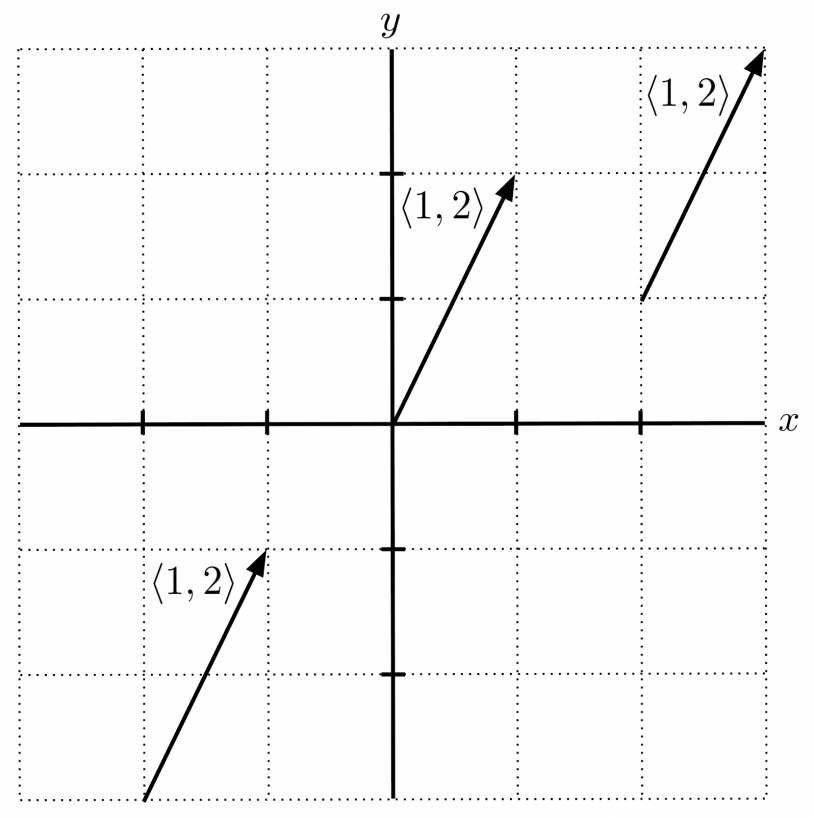Vectors can be added component-wise, and adding a sequence of vectors together yields a net displacement through all the vectors combined, starting each vector where the previous one ends.

\begin{align*} \left< 1,2 \right> + \left< -5,-1 \right> + \left< 1, -2 \right> &= \left< 1-5+1, 2-1-2 \right> \\ &= \left< -3, -1 \right> \end{align*}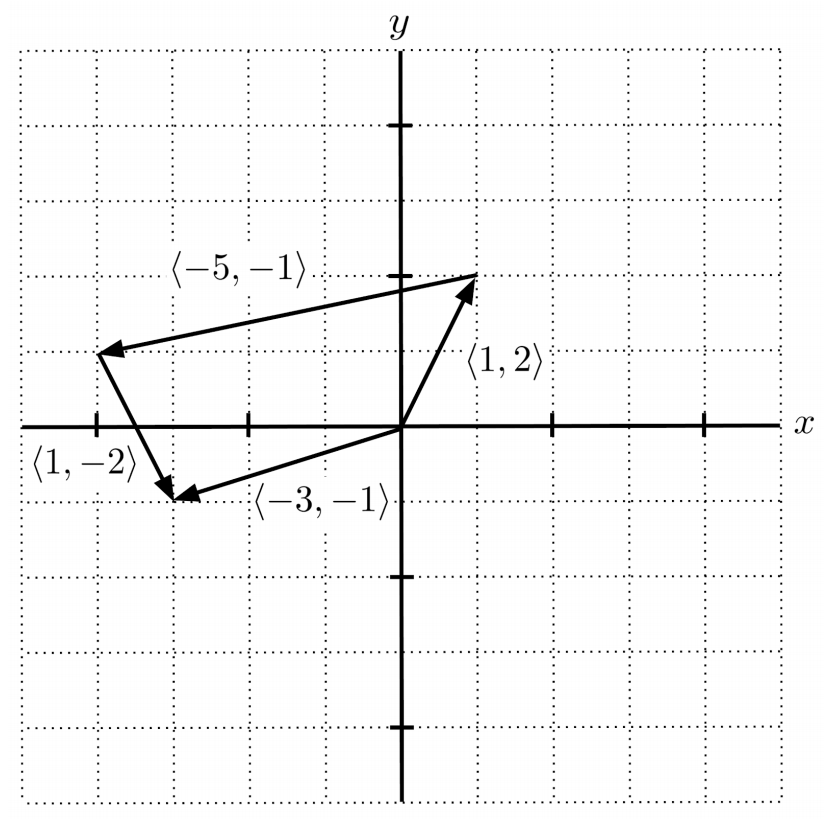## Scalars

Vectors can also be multiplied by regular numbers called scalars. Multiplying a vector by a scalar has the effect of rescaling a vector to become shorter or longer, depending on the magnitude of the scalar.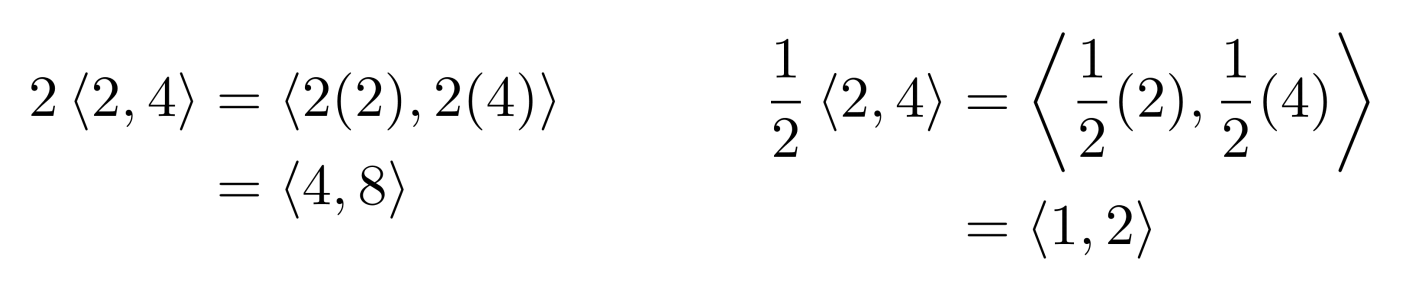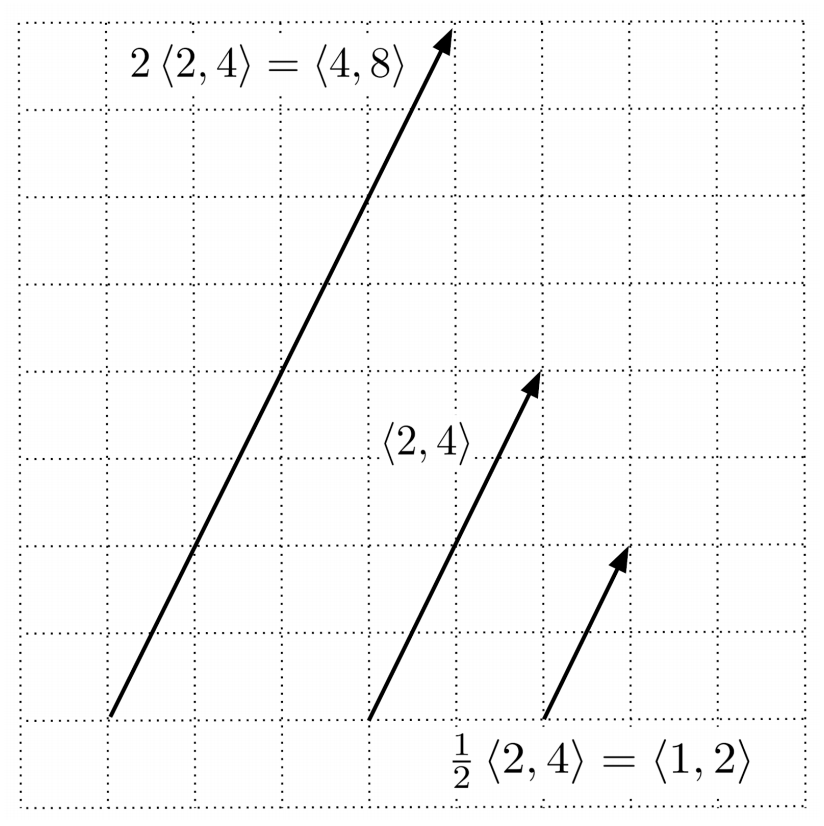If the scalar is negative, then the vector also flips direction, in addition to being rescaled by the magnitude of the scalar.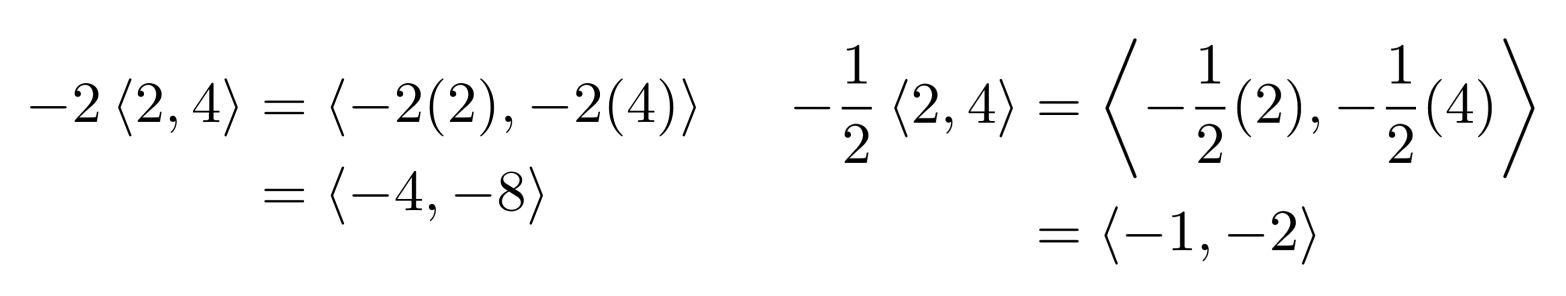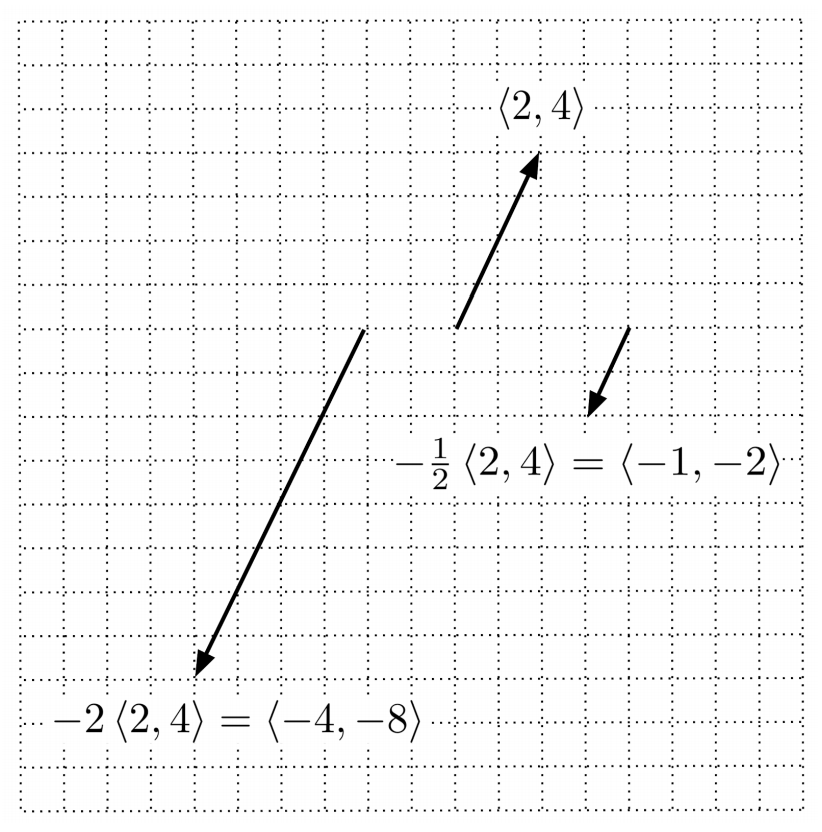## Norm of a Vector

In two dimensions, a vector’s length, called its norm, can be obtained using the Pythagorean theorem: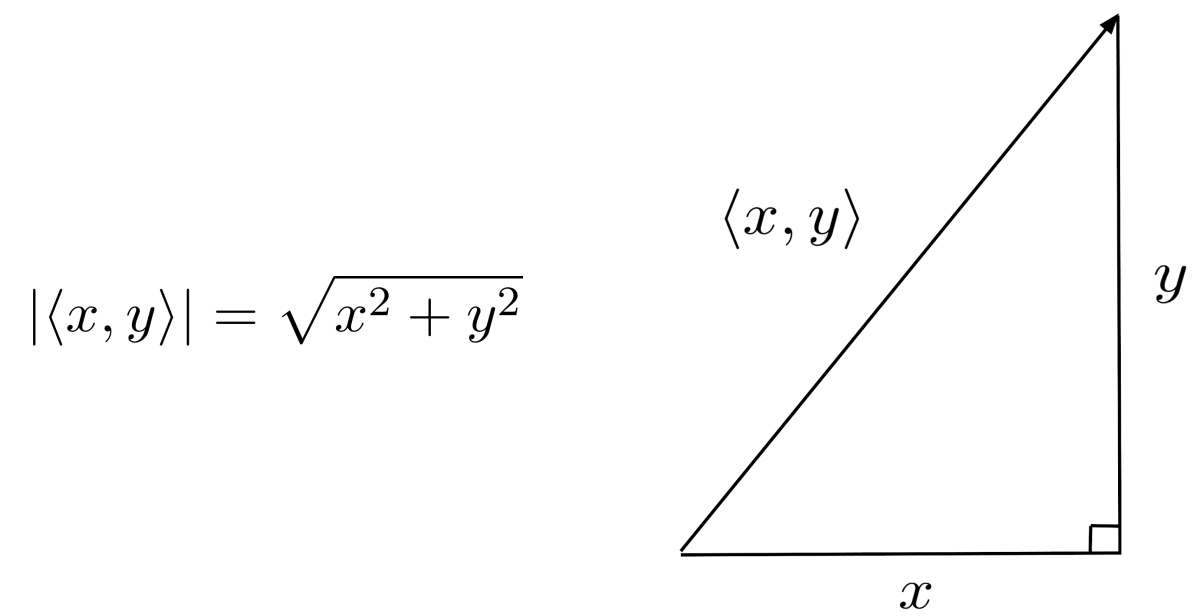In general, the norm of a vector can be computed by extending the Pythagorean theorem to higher dimensions.

\begin{align*} \left| \left< x_1, x_2, \ldots, x_n \right> \right| = \sqrt{x_1^2 + x_2^2 + \ldots + x_n^2} \end{align*}

To see that this definition of the norm is compatible with the idea that scalar multiplication rescales a vector, observe that the norm of a scaled vector is equal to the product of the scalar and the norm of the unscaled vector.

\begin{align*} \left| c \left< x_1, x_2, \ldots, x_n \right> \right| &= \left| \left< cx_1, cx_2, \ldots, cx_n \right> \right| \\ &= \sqrt{(cx_1)^2 + (cx_2)^2 + \ldots + (cx_n)^2} \\ &= \sqrt{c^2x_1^2 + c^2x_2^2 + \ldots + c^2x_n^2} \\ &= \sqrt{c^2(x_1^2 + x_2^2 + \ldots + x_n^2)} \\ &= \sqrt{c^2} \sqrt{x_1^2 + x_2^2 + \ldots + x_n^2} \\ &= c \left| \left< x_1, x_2, \ldots, x_n \right> \right| \end{align*}

## Algebra with Vectors

Lastly, expressions involving multiple vector operations follow the standard rules of arithmetic, and equations involving vector variables follow the standard rules of algebra.

\begin{align*} 2 \left( \left< 3,2 \right> - x \right) &= \left< 4,2 \right> \\ 2 \left< 3,2 \right> - 2x &= \left< 4,2 \right> \\ \left< 6,4 \right> - 2x &= \left< 4,2 \right> \\ - 2x &= \left< 4,2 \right> - \left< 6,4 \right> \\ - 2x &= \left< -2,-2 \right> \\ x &= -\frac{1}{2} \left< -2,-2 \right> \\ x &= \left< 1,1 \right> \end{align*}

We can also use algebra to solve for unknown components of vectors.

\begin{align*} \left| 2 \left< 3,2,x \right> \right| &= \left| \left< 1,2,3 \right> + \left< 5,4,1 \right> \right| \\ 2 \left| \left< 3,2,x \right> \right| &= \left| \left< 6,6,4 \right> \right| \\ \left| \left< 3,2,x \right> \right| &= \frac{1}{2} \left| \left< 6,6,4 \right> \right| \\ \left| \left< 3,2,x \right> \right| &= \left| \frac{1}{2} \left< 6,6,4 \right> \right| \\ \left| \left< 3,2,x \right> \right| &= \left| \left< 3,3,2 \right> \right| \\ \sqrt{3^2+2^2+x^2} &= \sqrt{3^2+3^2+2^2} \\ \sqrt{9+4+x^2} &= \sqrt{9+9+4} \\ \sqrt{13+x^2} &= \sqrt{22} \\ 13+x^2 &= 22 \\ x^2 &= 9 \\ x &= \pm 3 \end{align*}

## Exercises

For each function, list the dimensionalities of the input space and the output space. (You can view the solution by clicking on the problem.)

$1) \hspace{.5cm} f(x,y)=(x,y,x+y)$
Solution:
$\text{input dimension: } 2$
$\text{output dimension: } 3$

$2) \hspace{.5cm} f(x) = x^3$
Solution:
$\text{input dimension: } 1$
$\text{output dimension: } 1$

$3) \hspace{.5cm} f(x,y,z) = xyz$
Solution:
$\text{input dimension: } 3$
$\text{output dimension: } 1$

$4) \hspace{.5cm} f(x,y)=(xy, x^2y^2, \ldots, x^ny^n)$
Solution:
$\text{input dimension: } 2$
$\text{output dimension: } n$

$5) \hspace{.5cm} f(x_1, x_2, \ldots, x_m) = (x_1+x_2, x_2+x_3, \ldots, x_{m-1}+x_m)$
Solution:
$\text{input dimension: } m$
$\text{output dimension: } m-1$

$6) \hspace{.5cm} f(x_1, x_2, \ldots, x_m, y_1, y_2, \ldots, y_n) = \left( \frac{x_1+x_2+\ldots+x_m}{m}, \sqrt[n]{y_1y_2\ldots y_n} \right)$
Solution:
$\text{input dimension: } m+n$
$\text{output dimension: } 2$

Perform the indicated vector operations. (You can view the solution by clicking on the problem.)

$7) \hspace{.5cm} 3 \left< -1,2,1 \right> + \frac{1}{2} \left< 4,4,-2 \right>$
Solution:
$\left< -1,8,2 \right>$

$8) \hspace{.5cm} 4( \left< 1,2 \right> - \left< 3,2 \right> )$
Solution:
$\left< -8,0 \right>$

$9) \hspace{.5cm} |\left< 1,0,1,2,0 \right>| + |\left< 0,0,-4,4,-8 \right>|$
Solution:
$5\sqrt{6}$

$10) \hspace{.5cm} |\left< -1,1,0 \right> - \left< -1,3,-2 \right>|$
Solution:
$2\sqrt{2}$

$11) \hspace{.5cm} | \left< 1,2 \right> | (\left< 1,1,1,-1 \right> - \left< 1,-1,1,1 \right> )$
Solution:
$\left< 0,2\sqrt{5},0,-2\sqrt{5} \right>$

$12) \hspace{.5cm} \frac{\left< 12,4,-16,0,-5 \right> + \left< 3,1,-4,5,-5 \right>}{| \left< 0,3,4 \right>|}$
Solution:
$\left< 3,1,-4,1,-2 \right>$

Solve for the unknown variable.

$13) \hspace{.5cm} \left< 1,2 \right> + x = \left< 4,7 \right>$
Solution:
$x= \left< 3,5 \right>$

$14) \hspace{.5cm} \left< 1,7,-3,8,2 \right> =\left< -2,8,0,x,3 \right> - \left< -3,1,3,6,1 \right>$
Solution:
$x= 14$

$15) \hspace{.5cm} |\left< 3,x \right>| \left< 1,2,3 \right> = \left< 5,10,15 \right>$
Solution:
$x= \pm 4$

$16) \hspace{.5cm} \left< x+1, x-1, 3 \right> + \left< 0,1,2 \right> = \left< -1,-2,5 \right>$
Solution:
$x= -2$

$17) \hspace{.5cm} 3(x+\left< 1,-1,1,-1 \right>) = 2x$
Solution:
$x= \left< -1,1,-1,1 \right>$

$18) \hspace{.5cm} | \left< x,x,x \right> + \left< -1,0,1 \right> | = 2$
Solution:
$x= \pm \sqrt{ \frac{2}{3} }$

This post is part of a series.

Tags: